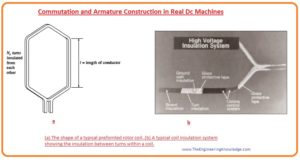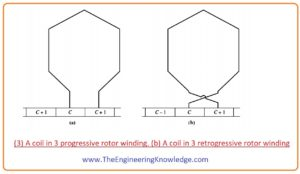Hello, fellows, I hope all of you are having fun in your life. In today’s tutorial, we will discuss the Commutation and Armature Construction in Real DC Machines. In DC machines there are many methods to join armature windings with the commutators. These methods of connection define the no of parallel paths for current in rotor, value of terminal voltage, no of commutators and their location. Commutation is processed by which ac voltage converted into the dc voltage. The working of dc machine is such that they produce ac voltages at their output terminals these ac voltages are converted into a dc voltage.

In today’s post, we will have a look at designing and arrangement of windings on a rotor that called armature windings and their linking with the commutators to ac to dc conversions. So let’s get started with Commutation and Armature Construction in Real Dc Machines.

#### Commutation and Armature Construction in Real DC Machines

Rotor Windings

• Irrespective of the method in which the windings are linked with commutators, mostly armature windings comprises of diamond-like arrangements of coils that are placed into the slots at the rotor.• Every coil comprises a number of turns of wires, every turn was covered with some insulating material and there is further insulation among the turns and from slots that used to insertion of windings.
• Every side of the turn is named as a conductor. You can use this given formula to find the number of conductors in armature windings.

Z=2CNc

• In this formula.
• Z is the no of a conductor in the rotor.
• C is the no of a coil in the rotor.
• Nc is no of turns per coils.
• The electrical angle of a coil is one eighty degrees, which means that one side of the windings is facing one pole its other side is facing a pole of other polarities.
• The pole is not at an angle of one eight degrees physically, but the polarity of the field will change moving from the first pole to second pole.
• The mathematical relation among mechanical and electrical angles can be written as.

øe =(P/2) øm

in this equation.

øe is the electrical angle.

P is no of poles.

øm is a mechanical angle.

• If the electrical angle is one eighty degrees then the voltage at any side of the coil’s conductor will be similar in magnitude but has reverse polarity.
• This type of windings is known as full pitch windings.
• If the electrical angle is less than the one eighty degrees than this type of windings is known as fractional pitch coil, if windings of the rotor are fractional pitch then these windings known as chorded windings.
• The quantity of chording is defined by some factor that is known as pitch factor p, which can be described as.

P =(electrical angle/1800) x 100%

• To enhance commutation in the windings of a rotor normally less quantity of chording is employed.
• Normally windings used in the rotor are 2 layer windings, that have in every coil two different windings are placed.
• One side of the windings coil is placed at bottom of its slot, and the second side is placed at the top of the slot.
• This type of arrangement needs the special technique to put placed coils in the slots of rotor.• One end of every coil is located at the bottom of its slot, and then all the bottom sides are in place, the other end of every coil is located at the top of its slot.
• In this style, all the windings are interlaced together, enhancing the mechanical strength and homogeneity of the concluding assembly.

#### Connections to the Commutator Segments

• After placing the windings in the rotor’s slots of dc machines next process is to link these windings with the commutator.
• There are numerous techniques that can be used for windings joining with the commutators and many arrangements of windings can be used for this process each has its own benefits and drawbacks.
• The space among the split rings or commutator parts that are connected with the 2 ends of windings are known as the pitch of commutator and denoted as yc.

#### Progressive windings

• In these arrangements of windings connection with the commutator is such that one end is connected with one commutator segment and another end of windings is connected to the next commutator section. (It simple word we can explain it that if the first commutator segment is ‘n’ and next is ‘n+1’ and windings are connected with these two).

#### Retrogressive winding

• In this type of windings arrangement, one end of windings is connected with one commutator segment and a second end with the previous commutator. We can understand it in simple words that if we connect one end with ‘n’ commutator segment and second with ‘n-1’ segment than it called retrogressive windings.
• The rotor of these two windings has a different rotation direction.• The windings at the rotor have different classifications rendering to plex.

#### Simplex windings:

• This winding has one complete winding wound on the rotor of the machine.

#### Duplex winding:

• This winding has 2 sets of windings wound on the rotor. In this windings every winding is linked with the commutator segment after leaving one, mean one winding is linked with the 1, 3, 5,7, etc, commutators and other will be linked 2,4,6,8 segment.

#### Triplex windings

• This winding has 3 sets of windings on the rotor and each is linked with the 3rd commutator segment
• In a simple word, if the rotor has more than one winding then these windings are known as multiplex windings.

That is the complete post on the Commutation and Armature Construction in Real DC Machines if you have any questions about this post ask in comments. See you in the next tutorial.